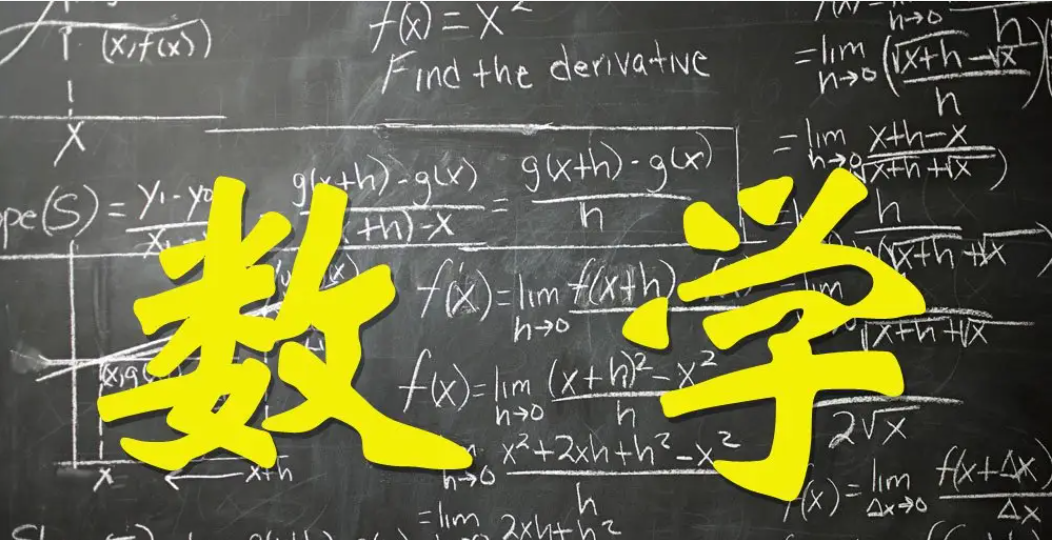# 2022年函授大专数学必考知识点大集合

2022年函授大专报考条件基本是考生须具有高中毕业文化程度且年满18周岁，成人大专考试科目有数学(文/理)、英语和语文，那么2022年成考数学怎么复习呢?

交集和并集

1.取集合A和集合B的公共部分，记作A∩B。

2.取集合A和集合B的全部元素，记作A∪B。

简单逻辑

1.充分条件：如果A成立，那么B成立，“A推出B，B不能推出A”。

2.必要条件：如果B成立，那么A成立，“B推出A，A不能推出B”。

3.充要条件：如果A→B，又有A←B，“A推出B，B推出A”。

函数部分

1.绝对值的不等式

绝对值不等式的解法:

|ax+b|

(当a<0的时候，不等号要改变方向

|ax+b|>c相当于解不等式ax+b>c或ax+b<-c

2.常见函数的定义域

3.函数的单调性

第一种方法用取值法:任取2个数x1，x2，且x1

若f(x1)f(x2)，则为减函数。

第二种方法用求导法(见后面)。

4.函数的奇偶性

令x=-x，若f(-x)=-f(x)，则f(x)为奇函数;

若f(-x)=f(x)，则f(x)为偶函数。

向量和直线

1.向量

设a=(x1,y1)b=(x2,y2)，则:

加法运算:a+b=(x1,y1)+(x2,y2)=(x1+x2,y1+y2)

减法运算:a-b=(x1,y1)-(x2y2)=(x1-x2:y1-y2)

数乘运算:ka=k(x1,y1)=(kx1,ky1)

内积运算:a*b=(x1,y1)(x2,y2)= x1x2 +y1y2

垂直向量:a⊥b= x1x2 +y1y2=0

平行向量:a//b= x1y2 +x2y1=0

2.直线方程的几种形式(记住其中一种就可以)

点斜式:y-yo=k(x-x0)，已知斜率k和某点坐标(xo,yo)

斜截式:y=kx+b，已知斜率k和在y轴的截距b

绝对值不等式的解法:

|ax+b|

(当a<0的时候，不等号要改变方向)

|ax+b|>c,相当于解不等式ax+b>c或ax+b<-c导数的应用

1.导数的几何意义

(1.几何意义:函数f(x)在点(x0,y0)处的导数值f'(x0)，即为f(x)在点(x0,y0)处切线的斜率。

(2.常用导数公式:c为常数

2.函数单调性

f'(x)>0则f(x)在(a，b)内严格单调增加

f'(x)<0则f(x)在(a，b)内严格单调减少。

3.函数的极值、最大值、最小值

f'(x)=0的点----函数f(x)的驻点。设为x0

(1.若x< x0时，f'(x)>0;x> x0时，f'(x)<0，则f(x0)为f(x)的极大值点。

(2.若x

(3.如果f'(x)在x0的两侧的符号相同，那么f(x0)不是极值点。

(4.极值和端点的函数值中最大和最小的就是最大值和最小值。

2.部分稿件来源于网络，如有不实或侵权，请联系我们沟通解决。最新官方信息请以各省教育考试院及各教育官网为准！
2023年湖南成人高考人手一份助学资料包，限时免费领取！"2022年函授大专数学必考知识点大集合" 相关文章推荐